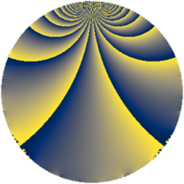# Properties

 Label 2475.1.tLevel $2475$ Weight $1$ Character orbit 2475.t Rep. character $\chi_{2475}(274,\cdot)$ Character field $\Q(\zeta_{6})$ Dimension $4$ Newform subspaces $1$ Sturm bound $360$ Trace bound $0$

# Related objects

## Defining parameters

 Level: $$N$$ $$=$$ $$2475 = 3^{2} \cdot 5^{2} \cdot 11$$ Weight: $$k$$ $$=$$ $$1$$ Character orbit: $$[\chi]$$ $$=$$ 2475.t (of order $$6$$ and degree $$2$$) Character conductor: $$\operatorname{cond}(\chi)$$ $$=$$ $$495$$ Character field: $$\Q(\zeta_{6})$$ Newform subspaces: $$1$$ Sturm bound: $$360$$ Trace bound: $$0$$

## Dimensions

The following table gives the dimensions of various subspaces of $$M_{1}(2475, [\chi])$$.

Total New Old
Modular forms 36 12 24
Cusp forms 12 4 8
Eisenstein series 24 8 16

The following table gives the dimensions of subspaces with specified projective image type.

$$D_n$$ $$A_4$$ $$S_4$$ $$A_5$$
Dimension 4 0 0 0

## Trace form

 $$4 q + 2 q^{4} + 2 q^{9} + O(q^{10})$$ $$4 q + 2 q^{4} + 2 q^{9} - 2 q^{11} - 2 q^{16} + 2 q^{31} + 4 q^{36} - 4 q^{44} + 2 q^{49} - 2 q^{59} - 4 q^{64} + 4 q^{69} - 4 q^{71} - 2 q^{81} - 8 q^{89} + 2 q^{99} + O(q^{100})$$

## Decomposition of $$S_{1}^{\mathrm{new}}(2475, [\chi])$$ into newform subspaces

Label Dim $A$ Field Image CM RM Traces $q$-expansion
$a_{2}$ $a_{3}$ $a_{5}$ $a_{7}$
2475.1.t.a $4$ $1.235$ $$\Q(\zeta_{12})$$ $D_{3}$ $$\Q(\sqrt{-11})$$ None $$0$$ $$0$$ $$0$$ $$0$$ $$q-\zeta_{12}q^{3}-\zeta_{12}^{4}q^{4}+\zeta_{12}^{2}q^{9}-\zeta_{12}^{2}q^{11}+\cdots$$

## Decomposition of $$S_{1}^{\mathrm{old}}(2475, [\chi])$$ into lower level spaces

$$S_{1}^{\mathrm{old}}(2475, [\chi]) \cong$$ $$S_{1}^{\mathrm{new}}(495, [\chi])$$$$^{\oplus 2}$$